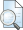## PENGGUNAAN BILANGAN PRIMA DALAM ALGORITMA KUNCI PUBLIK RSA, RABIN, DAN ELGAMAL PADA KRIPTOGRAFI

Arsyad, Abdurrahim (2011) PENGGUNAAN BILANGAN PRIMA DALAM ALGORITMA KUNCI PUBLIK RSA, RABIN, DAN ELGAMAL PADA KRIPTOGRAFI. Other thesis, University of Muhammadiyah Malang.Preview
Text
PENGGUNAAN_BILANGAN_PRIMA_DALAM_ALGORITMA_KUNCI_PUBLIK_RSA.pdf - Published Version

## Abstract

Cryptography is a technique to encrypt messages which using mathematical sciences in it. Mathematical sciences who exploited them are prime numbers with all the properties of round numbers, the modulo arithmetic, powers, greatest common divisor, square roots, etc. Cryptography has three important elements. Encryption, decryption, and key. Encryption is the process of forming understandable messages (plaintext) into the message that is not understandable (ciphertext). Decryption is the process of return messages that are not understandable (ciphertext) into an understandable message (plaintext). The key is a tool used for encryption and decryption process. Cryptography also have the encryption algorithms that are divided into symmetric algorithms and asymmetric algorithms (public key algorithm). Asymmetric algorithms include RSA, Rabin, and ElGamal. RSA, Rabin, and ElGamal using prime numbers as an initial benchmark calculation of encryption, and includes the process of encryption and decryption. In this thesis focused on the use of triple-digit prime and its calculation on public key algorithm RSA, Rabin, and ElGamal. Keywords : prime numbers, cryptography, algorithms, RSA, Rabin, ElGamal, encryption, decryption, and key.

Item Type: Thesis (Other) L Education > L Education (General) Faculty of Teacher Training and Education > Department of Mathematics Education (84202) Anwar Jasin 20 Feb 2012 06:22 20 Feb 2012 06:22 http://eprints.umm.ac.id/id/eprint/114

### Actions (login required)View Item